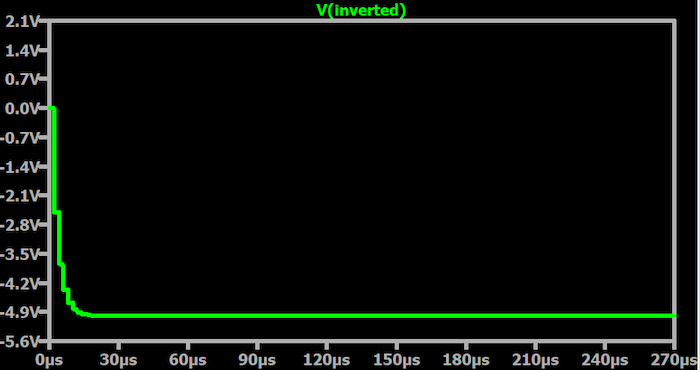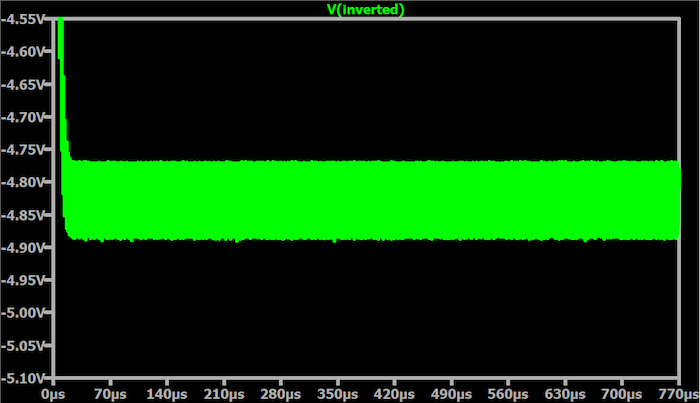Technical Article

# Analyzing a Negative Voltage Charge Pump in LTspice—Source and Load Resistance

May 14, 2023 by Robert Keim

## Learn about using LTspice simulations to provide important insight into the performance of a switched-capacitor voltage-inverting power supply.

Previously, I wrote an article that explained the basic principles of negative voltage, and I continued this theme with an LTspice lab that used simulations to elucidate negative voltage as something that occurs in and is produced by electrical circuits. As part of this LTspice lab, I'll also introduce a circuit topology that can produce a negative voltage that is stable and capable of supplying current to other components.

In this new series of articles, I would like to take a more detailed look at the functionality of this negative voltage circuit, with the objective of enhancing our understanding of how a real-life switched-capacitor power supply and power supplies, in general, can be optimized.

### Recap: Negative Voltage From Capacitors and Switches

Before diving in, let's look at Figure 1, which shows the charge pump circuit that I previously presented in the last negative voltage article.##### Figure 1. Example charge pump circuit.

In the circuit schematic, V1 produces the input voltage, and V2 generates a 500 kHz square wave that controls all four switches. Due to the different resistance values assigned to the on and off states in the SW1 and SW2 models, S1 and S3 are on when S2 and S3 are off, and vice versa. The source voltage charges capacitor C1 when S1 and S3 allow current flow, then all four switches change state, such that C1 discharges into the right side of the circuit.

Next, C2 acquires a potential difference equal to the V1 source voltage, but since the higher-voltage terminal of C2 is grounded, the lower-voltage terminal must shift into the negative voltage region. Thus, the voltage at the INVERTED node is equal to negative V(SOURCE). In other words, VOUT = –VIN.

The plot below (Figure 2) shows the output voltage pumping down to and then remaining at –VIN.### Understanding the Effect of Load Resistance

Perhaps you’re wondering if the switched-capacitor circuit is too good to be true. Just two capacitors, four switches, and a square wave? That’s all we need to generate a nicely regulated negative voltage supply rail? Well, not quite; this circuit is not actually a voltage regulator.

It’s not a voltage regulator because it lacks something that is central to the operation of both linear regulators and switch-mode regulators: a feedback subsystem. Regulators maintain stable, predictable supply voltages by monitoring the output and compensating for load variations through negative feedback.

Our switched-capacitor charge pump doesn’t have any sort of negative feedback control system, and consequently, a decrease in load resistance will cause a corresponding decrease in the output voltage. This occurs because the output network is essentially a voltage divider. With that in mind, we have the full –VIN at the output when RLOAD = 100 kΩ only because 100 kΩ is much higher than the source resistance (ROUT) of the charge pump. As RLOAD decreases toward ROUT, voltage is divided more equally between these two resistances, and thus the output voltage (i.e., the voltage across RLOAD) decreases.

You can also think about this in terms of load current. Let’s say that the operation of the load circuitry changes such that the supply must deliver more current (this is electrically equivalent to a reduction in RLOAD). When this occurs, more current flows through ROUT, more voltage is dropped across ROUT, and a smaller proportion of the input potential difference is available at the output node.

### Simulating Variable Load Resistance in LTspice

We can use a .step text command, placed right onto the LTspice schematic, to visually assess the effect of varying RLOAD:

.step PARAM LOAD list 100k 10k 1k 100 10


This statement will cause the simulation to run once for each value in the list attached to the variable LOAD. We want to assign these values to the RLOAD component, and we accomplish that by using {LOAD} (don’t forget the curly brackets) in the component-value field (shown in Figure 3):##### Figure 3. A section of the charge pump circuit showing the Rload {LOAD}.

The result can be seen in Figure 4 below.##### Figure 4. The resulting simulation results for the example charge pump circuit.

The three highest resistance values (100 kΩ, 10 kΩ, 1 kΩ) all lead to similar performance, and the traces corresponding to these three values are almost indistinguishable. However, at 100 Ω (the beige trace), we begin to notice a decrease in output voltage, and at 10 Ω (the green trace), the decrease is rather severe.

(I’m sure you also noticed that voltage ripple increases significantly as load resistance decreases. We’ll discuss that in Part 2.)

### Evaluating Output Voltage for Application Feasibility

Simulations like this one help us to determine whether the circuit will maintain adequate output voltage for a given application. Let’s say that we need a negative voltage to power a component with a supply requirement of –5 V ± 0.3 V; the minimum acceptable voltage magnitude, in this case, is 4.7 V. Using our previous results as a starting point, we create another simulation (Figure 5) with RLOAD values that will bring us near the relevant voltage threshold.

.step PARAM LOAD list 300 100 70 40##### Figure 5.  Simulation results where the RLOAD brings us near the relevant voltage threshold.

Our results suggest that the minimum safe RLOAD is a bit below 70 Ω. We’ll call it 65 Ω. A single-run simulation with RLOAD = 65 Ω confirms that we’re (theoretically) within the acceptable range, which can be seen below in Figure 6.##### Figure 6. A single simulation result with RLOAD =  65 Ω.

Ohm’s law tells us that the load current with RLOAD = 65 Ω will be approximately 74 mA—you can confirm this via simulation if you like. Thus, we conclude that if the total load current is less than 74 mA, the charge pump will be able to maintain adequate negative supply voltage for the component in question.

All in all, we examined some interesting details of an LTspice switched-capacitor charge pump, noting that the circuit is not a voltage regulator and using .step simulations to determine load-current capabilities. In the next article, we’ll take a closer look at output ripple.